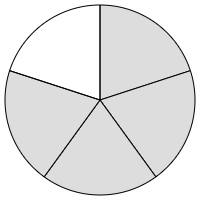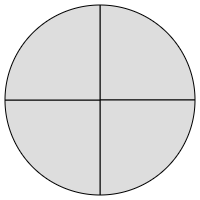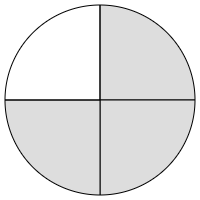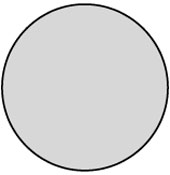# Math help from the Learning Centre

This guide provides useful resources for a wide variety of math topics. It is targeted at students enrolled in a math course or any other Centennial course that requires math knowledge and skills.

## Forms of Fractions

A proper fraction is a fraction whose numerator is smaller than its denominator.

For example: $$\dfrac{1}{2} , \dfrac{4}{5} , \dfrac{3}{7}$$

Consider the proper fraction $$\dfrac{4}{5}$$.
It can be visualised as:An improper fraction is a fraction whose numerator is greater than its denominator.

For example: $$\dfrac{3}{2} , \dfrac{7}{4} , \dfrac{9}{5}$$

Consider the improper fraction $$\dfrac{7}{4}$$.
It can be visualised as:A mixed fraction or mixed number is a combination of a whole number and a proper fraction.

For example: $$3\dfrac{1}{2} , 5\dfrac{2}{5} , 1\dfrac{3}{4}$$

The mixed fraction $$1\dfrac{3}{4}$$ means there is one whole part plus $$\dfrac{3}{4}$$ of a whole part.
In fact, $$1\dfrac{3}{4} = 1 + \dfrac{3}{4}$$ and can be visualised as:Notice that the shaded region in the circles representing $$\dfrac{7}{4}$$ and $$1\dfrac{3}{4}$$ are the same!
In fact, $$1\dfrac{3}{4} = \dfrac{7}{4}$$.
They are different ways of representing the same thing!

## Switching Between Forms: Mixed and Improper Fractions

To switch from mixed to improper:

1. Multiply the whole number by the denominator of the proper fraction
2. Add to the numerator of the proper fraction.

Examples

• $$4\frac{2}{3} = \dfrac{4\times3+2}{3} = \dfrac{12+2}{3} = \dfrac{14}{2}$$

• $$5\frac{1}{4} = \dfrac{5\times4+1}{4} = \dfrac{20+1}{4} = \dfrac{21}{4}$$

• $$2\frac{3}{7} = \dfrac{2\times7+3}{7} = \dfrac{14+3}{7} = \dfrac{17}{7}$$

To switch from improper to mixed:

1. Use long division to divide the denominator into the numerator.
2. We will use the Quotient and Remainder to write our Mixed Fraction.

The improper fraction in mixed form is:      $$\text{Quotient}\dfrac{\text{Remainder}}{\text{Denominator}}$$

Example

Suppose we want to change $$\dfrac{21}{4}$$ to a mixed fraction.

$$21$$ divided by $$4$$ is $$5$$ with remainder $$1$$.

So:

$$\dfrac{21}{4} = 5\frac{1}{4}$$

1. Make sure the denominators are the same.**

3. Simplify the fraction if necessary.
(Done by dividing numerator and denominator by their Greatest Common Factor (GCF)).

**If they are not, this can be done by rewriting one/both of the fractions by multiplying both numerator and denominator by the same value.

Example
$$\dfrac{1}{2}$$ is the same as $$\dfrac{2}{4}$$ because $$\dfrac{1}{2} = \dfrac{1\times 2}{2\times 2} = \dfrac{2}{4}$$

For simplicity, we aim to multiply both fractions separately to ensure each denominator becomes the least common multiple (LCM) of the two original denominators. See Example 2.

Suppose we want to add: $$\dfrac{3}{12} + \dfrac{5}{12}$$.

The denominators are the same, so we add the numerators and simplify:

\begin{align} \dfrac{3}{12}+\dfrac{5}{12} &= \dfrac{8}{12} \\ &= \dfrac{8 \div 4}{12 \div 4} \\ &= \dfrac{2}{3} \end{align}

The above calculation can be visualised as:Suppose we want subtract: $$\dfrac{2}{5} - \dfrac{1}{4}$$.

The denominators are not the same so we must rewrite one/both of the fractions.

The least common multiple of $$5$$ and $$4$$ is $$20$$, so we need to multiply each fraction above and below so that their denominator becomes $$20$$.

\begin{align} \dfrac{2}{5} &= \dfrac{2\times4}{5\times4} \\ &= \dfrac{8}{20}   \end{align}

and

\begin{align} \dfrac{1}{4} &= \dfrac{1\times5}{4\times5} \\ &= \dfrac{5}{20}   \end{align}

Now that the denominators are the same, the fractions can be subtracted as usual:

\begin{align} \dfrac{2}{5}-\dfrac{1}{4} &= \dfrac{8}{20}-\dfrac{5}{20} \\ &= \dfrac{3}{20} \end{align}

The above calculation can be visualised as:## Multiplying/Dividing Fractions

To multiply fractions:

1. Multiply the numerators together.
2. Multiply the denominators together.
3. As always, simplify if necessary.

In other words, remember the rule "Top by top, Bottom by bottom".

Example

\begin{align} \dfrac{3}{5} \times \dfrac{2}{7} &= \dfrac{3\times2}{5\times7} \\ &= \dfrac{6}{35} \end{align}

To divide fractions:

1. Change the division symbol to a multiplication symbol.
2. Flip the divisor.
The divisor is the dividing fraction in this case, like $$\frac{1}{2}$$ in $$\frac{3}{4} \div \frac{1}{2}$$
3. Perform the multiplication, and as always, simplify if necessary.

In other words, remember the rule "Keep, Change, Flip".

Example

\begin{align} \dfrac{2}{9} \div \dfrac{3}{4} &= \dfrac{2}{9} \times \dfrac{4}{3} \qquad \qquad \qquad \text{(1)} \\ &= \dfrac{2\times4}{9\times3} \\ &= \dfrac{8}{27} \end{align}

(1) Keep the $$\dfrac{2}{9}$$ , Change the division to multiplication, Flip the $$\dfrac{3}{4}$$